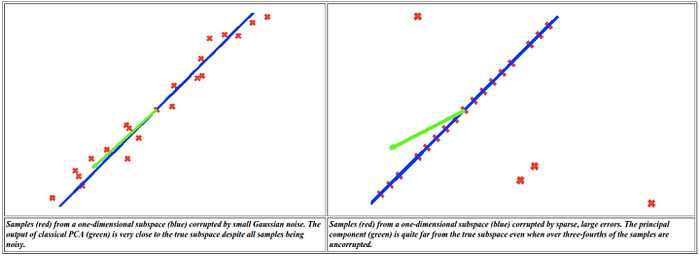# Robust Principal Component Analysis via ADMM in Python

Principal Component Analysis (PCA) is an effective tool for dimensionality reduction, transforming high dimensional data into a representation that has fewer dimensions (although these dimensions are not from the original set of dimensions). This new set of dimensions captures the variation within the high dimensional dataset. How do you find this space? Well, PCA is equivalent to determining the breakdown M = L + E, where L is a matrix that has a small number of linearly independent vectors (our dimensions) and E is a matrix of errors (corruption in the data). The matrix of errors, E, has been minimized. One assumption in this optimization problem, though, is that our corruption, E, is characterized by Gaussian noise .Sparse but large errors affect the recovered low-dimensional space. From: Introduction to RPCA

Robust PCA  is a way to deal with this problem when the corruption may be arbitrarily large, but the errors are sparse. The idea is to find the breakdown M = L + S + E, where we now have S, a matrix of sparse errors, in addition to L and E. This has been shown to be an effective way for extracting the background of images (the static portion that has a lower dimensionality) from the foreground (the sparse errors). I’ve used this in my own work to extract foreground dolphins from a pool background (I used the ALM method which only provides L and S).

Robust PCA can be solved exactly as a convex optimization problem, but the computational constraints associated with high dimensional data make exact solutions impractical. Instead, there exist fast approximation algorithms. These techniques include the inexact augmented Lagrange multiplier (ALM) method  and the alternating direction method of multipliers (ADMM) method .

For the most part, these approximation algorithms exist in MATLAB. There have been a few translations of these algorithms into Python. Shriphani Palakodety (code) and Deep Ganguli (code) both translated the Robust Principal Component Pursuit algorithm, Nick Birnie (code) translated the ALM method, and Kyle Kastner (code) translated the Inexact ALM method. There also exists Python bindings for an RPCA implementation in a linear algebra and optimization library called Elemental.

Since the ADMM method is supposed to be faster than the ALM method (here on slide 47), I thought it would be a good idea to translate this code to a working Python version. My translation, Robust Principal Component Analysis via ADMM in Python, implements the MATLAB code found here (along with [a] and [b]). In addition to porting this code to Python, I altered the update equations to use multiple threads to speed up processing.

## References

1. Lin, Chen, Ma (2010). The augmented lagrange multiplier method for exact recovery of corrupted low-rank matrices.
2. Candès, Li , Ma, and Wright (2011) Robust Principal Component Analysis?